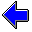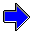Java Security Settings:
This web page employs Java, which requires specific security settings for correct operation.
If the applets on this page do not run correctly, consult the Virtual Chemistry Experiments FAQ
or the Physlet Physics web site for establishing the correct security settings.

# Chemical Kinetics

## Integrated Rate Laws

### Concepts

The differential rate law describes how the rate of reaction varies with the concentrations of various species, usually reactants, in the system. The rate of reaction is proportional to the rates of change in concentrations of the reactants and products; that is, the rate is proportional to a derivative of a concentration.

To illustrate this point, consider the reaction

A   →   B

The rate of reaction, r, is given by

 r = - d [A]d t

Suppose this reaction obeys a first-order rate law:

r = k [A]

This rate law can also be written as

 r = - d [A]d t = k [A]

This equation is a differential equation that relates the rate of change in a concentration to the concentration itself. Integration of this equation produces the corresponding integrated rate law, which relates the concentration to time. When you viewed concentration-time curves in previous pages, you viewed the integrated rate laws.

 d [A][A] = - k d t

At t = 0, the concentration of A is [A]0. The integrated rate law is thus

[A] = [A]0 e- k t

Experimentally one almost always measures how the concentration of a reactant or product changes as the reaction progresses. In the previous page, you saw how the derivative of the concentration-time curve can be used to determine the differential rate law for a reaction. While this approach might work for simple systems with numerically exact data, this method for determining a rate law does not work well in practice. The experimental data suffers from random error and frequently one only collects points infrequently. Consequently it is difficult or impossible to determine the slope accurately.

A much better practical approach is to make characteristic kinetics plots. For each integrated rate law, there is a characteristic plot that can be created which will produce a straight line. These characteristic plots are presented in the table shown below; species A is a reactant in the chemical reaction.

Reaction Order Differential Rate Law Integrated Rate Law Characteristic
Kinetic Plot
Slope of
Kinetic Plot
Units of
Rate Constant
Zero
 - d [A]d t = k
[A] = [A]0 - k t [A] vs t - k mole L-1 sec-1
First
 - d [A]d t = k [A]
[A] = [A]0 e- k t ln [A] vs t - k sec-1
Second
 - d [A]d t = k [A]2
 [A] = [A]01 + k t [A]0
1/[A] vs tk L mole-1 sec-1

The series of three graphs shown below illustrate the use of the characteristic kinetic plots. The graph on the left shows [A] vs t plots for a zero-order (red line), first-order (green line), and second-order (blue line) reaction. The graph in the middle shows ln [A] vs t plots for each reaction order, and the graph on the right shows 1/[A] vs t plots for each reaction order.

Notice that for each characteristic kinetic plot, a specific rate law shows a straight line. In the [A] vs t plot, only the zero-order reaction (red line) produces a straight line; the other lines curve. In the ln [A] vs t plot, only the first-order reaction (green line) produces a straight line, and in the 1/[A] vs t plot, only the second-order reaction (blue line) produces a straight line.

### Experiment

Objectives

• Determine the rate law for a chemical reaction.
• Determine the rate constant for a chemical reaction.

Consider the following reaction between the persulfate ion and the iodide ion:

S2O82- (aq) + 3 I - (aq)   →   2 SO42- (aq) + I3- (aq)

In this chemical system, the only species that absorbs visible light is the triiodide ion. Spectrophotometry can be employed to determine the concentration of triiodide ion, which with knowledge of the initial concentration of iodide ion permits the concentration of iodide ion to be determined at any point in time.

In this stopped-flow experiment, one syringe contains a 0.100 M solution of iodide ion and the other syringe contains a 0.100 M solution of persulfate ion. The two solutions are mixed in a 3:1 ratio, so that there is a stoichiometric amount of iodide and persulfate ions in the reaction solution. As the reaction is occurring, the concentration of iodide is plotted versus time in the left graph.

This reaction is expected to follow a generic rate law of the form

r = k [S2O82-]a [I -]b

This expression indicates that the reaction is of order a with respect to persulfate ion and order b with respect to iodide ion.
The overall order of the reaction is a + b. Because iodide and persulfate ion are present in a stoichiometric ratio throughout the reaction,

[I -] = 3 [S2O82-]

Substituting this expression into the general rate expression, one obtains the effective rate law

r = ko [I -]n

where n = a + b is the overall order of the reaction and the observed rate constant ko = k / 3a.

Run the stopped-flow experiment. After the reaction is complete, prepare kinetic plots for the zero-, first-, and second-order reactions. In the kinetics plots, the data is plotted as red points and the line-of-best-fit is plotted in blue. Use the kinetics plots to determine the rate law for this reaction (that is, determine the overall order of the reaction, n). The slope of the kinetic plot can be used to determine the rate constant ko for the reaction.

slope =   intercept =Differential Rate Laws                     Half-LifeChemical Kinetics Home PageVirtual Chemistry Home Page

IntegratedRateLaws.html version 2.1
© 2000-2014 David N. Blauch